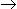# Aptitude - Numbers

## Why Aptitude Numbers?

In this section you can learn and practice Aptitude Questions based on "Numbers" and improve your skills in order to face the interview, competitive examination and various entrance test (CAT, GATE, GRE, MAT, Bank Exam, Railway Exam etc.) with full confidence.

## Where can I get Aptitude Numbers questions and answers with explanation?

IndiaBIX provides you lots of fully solved Aptitude (Numbers) questions and answers with Explanation. Solved examples with detailed answer description, explanation are given and it would be easy to understand. All students, freshers can download Aptitude Numbers quiz questions with answers as PDF files and eBooks.

## Where can I get Aptitude Numbers Interview Questions and Answers (objective type, multiple choice)?

Here you can find objective type Aptitude Numbers questions and answers for interview and entrance examination. Multiple choice and true or false type questions are also provided.

## How to solve Aptitude Numbers problems?

You can easily solve all kind of Aptitude questions based on Numbers by practicing the objective type exercises given below, also get shortcut methods to solve Aptitude Numbers problems.

### Exercise :: Numbers - General Questions

1.

Which one of the following is not a prime number?

 A. 31 B. 61 C. 71 D. 91

Explanation:

91 is divisible by 7. So, it is not a prime number.

2.

(112 x 54) = ?

 A. 67000 B. 70000 C. 76500 D. 77200

Explanation:

 (112 x 54) = 112 x104 = 112 x 104 = 1120000 = 70000 2 24 16

3.

It is being given that (232 + 1) is completely divisible by a whole number. Which of the following numbers is completely divisible by this number?

 A. (216 + 1) B. (216 - 1) C. (7 x 223) D. (296 + 1)

Explanation:

Let 232 = x. Then, (232 + 1) = (x + 1).

Let (x + 1) be completely divisible by the natural number N. Then,

(296 + 1) = [(232)3 + 1] = (x3 + 1) = (x + 1)(x2 - x + 1), which is completely divisible by N, since (x + 1) is divisible by N.

4.

What least number must be added to 1056, so that the sum is completely divisible by 23 ?

 A. 2 B. 3 C. 18 D. 21 E. None of these

Explanation:

``` 23) 1056 (45
92
---
136
115
---
21
---

Required number = (23 - 21)
= 2.
```

5.

1397 x 1397 = ?

 A. 1951609 B. 1981709 C. 18362619 D. 2031719 E. None of these

Explanation:

 1397 x 1397 = (1397)2 = (1400 - 3)2 = (1400)2 + (3)2 - (2 x 1400 x 3) = 1960000 + 9 - 8400 = 1960009 - 8400 = 1951609.

6.

How many of the following numbers are divisible by 132 ?
264, 396, 462, 792, 968, 2178, 5184, 6336

 A. 4 B. 5 C. 6 D. 7

Explanation:

132 = 4 x 3 x 11

So, if the number divisible by all the three number 4, 3 and 11, then the number is divisible by 132 also.

26411,3,4 (/)

39611,3,4 (/)

46211,3 (X)

79211,3,4 (/)

96811,4 (X)

217811,3 (X)

51843,4 (X)

633611,3,4 (/)

Therefore the following numbers are divisible by 132 : 264, 396, 792 and 6336.

Required number of number = 4.

7.

(935421 x 625) = ?

 A. 575648125 B. 584638125 C. 584649125 D. 585628125

Explanation:

 935421 x 625 = 935421 x 54 = 935421 x104 2

 = 935421 x 104 = 9354210000 24 16

= 584638125

8.

The largest 4 digit number exactly divisible by 88 is:

 A. 9944 B. 9768 C. 9988 D. 8888 E. None of these

Explanation:

```Largest 4-digit number = 9999

88) 9999 (113
88
----
119
88
----
319
264
---
55
---

Required number = (9999 - 55)
= 9944.
```

9.

Which of the following is a prime number ?

 A. 33 B. 81 C. 93 D. 97

Explanation:

Clearly, 97 is a prime number.

10.

What is the unit digit in {(6374)1793 x (625)317 x (341491)}?

 A. 0 B. 2 C. 3 D. 5

Explanation:

Unit digit in (6374)1793 = Unit digit in (4)1793

= Unit digit in [(42)896 x 4]

= Unit digit in (6 x 4) = 4

Unit digit in (625)317 = Unit digit in (5)317 = 5

Unit digit in (341)491 = Unit digit in (1)491 = 1

Required digit = Unit digit in (4 x 5 x 1) = 0.

#### Current Affairs 2022

Interview Questions and Answers